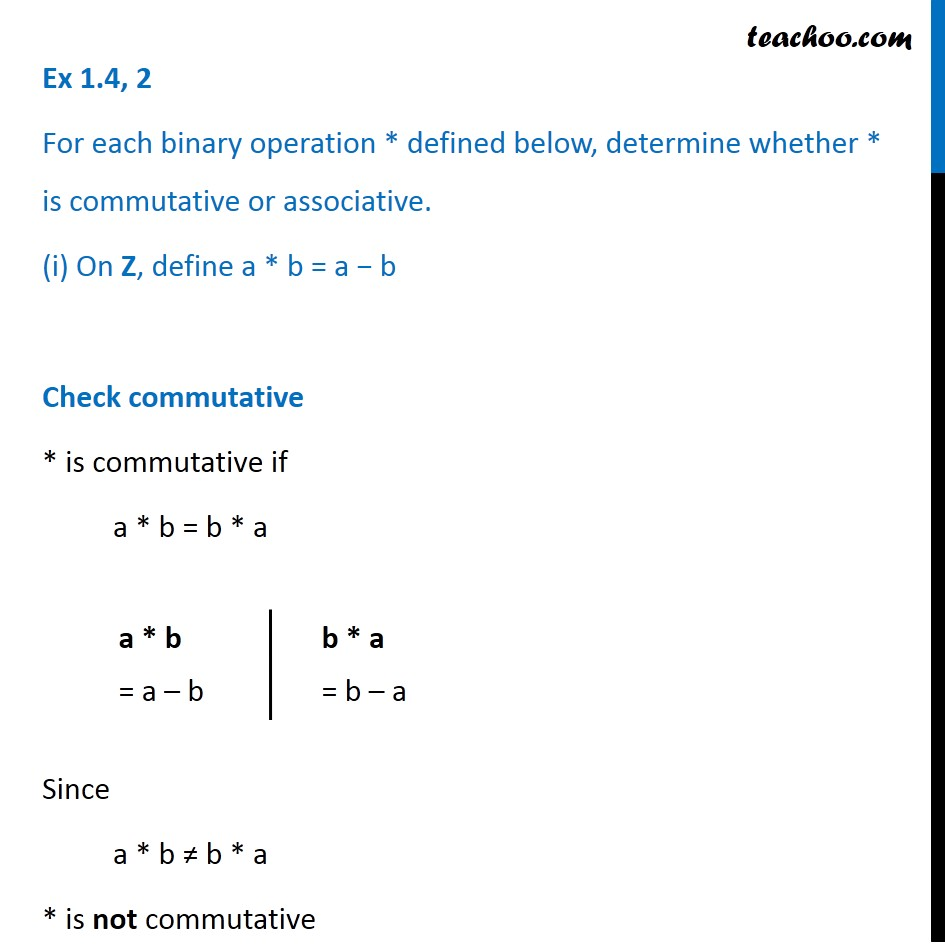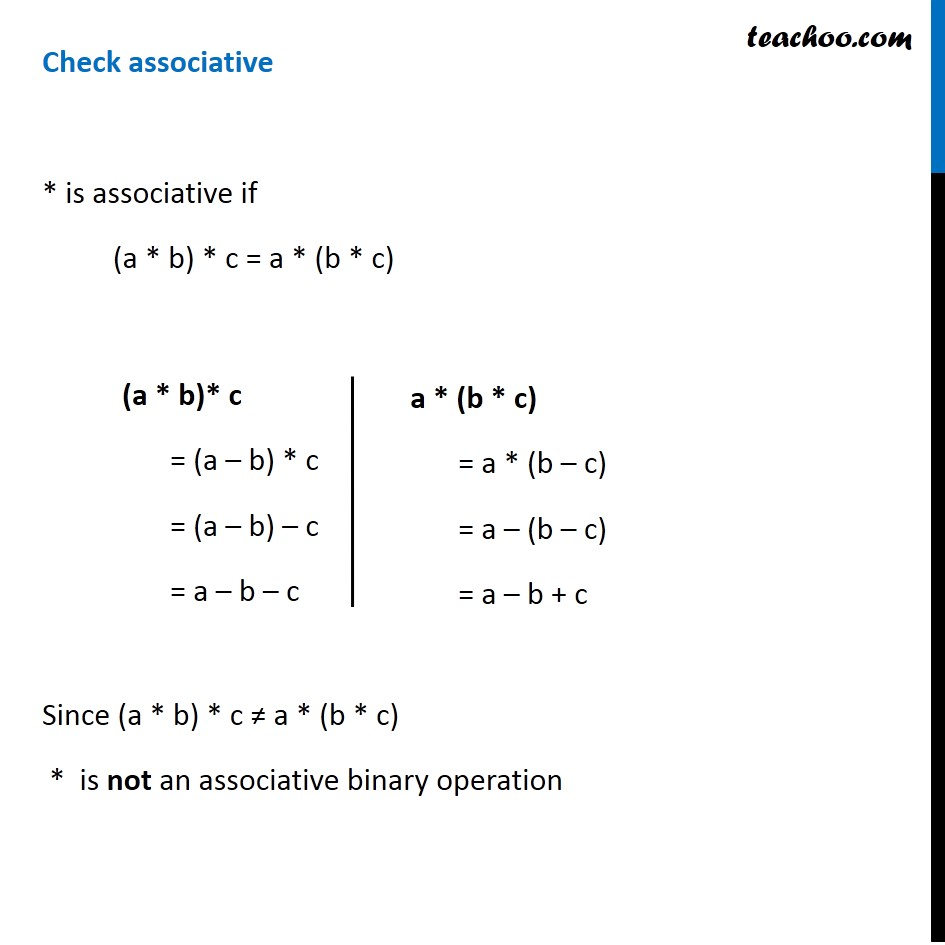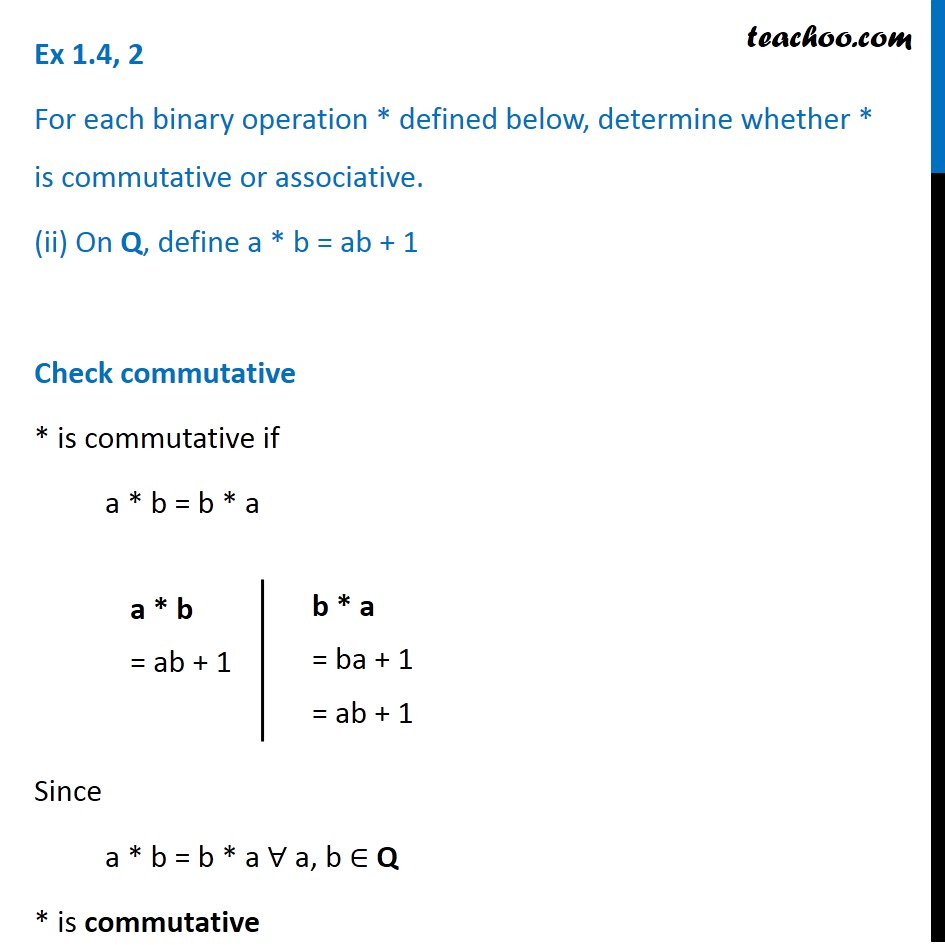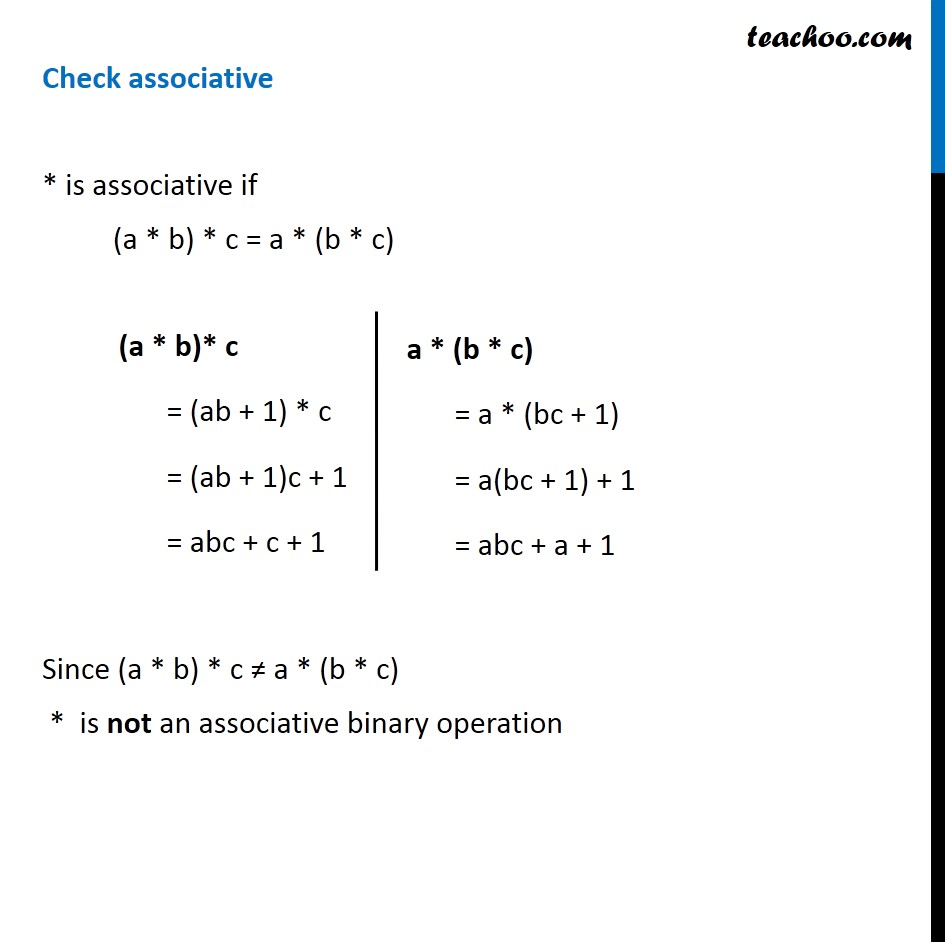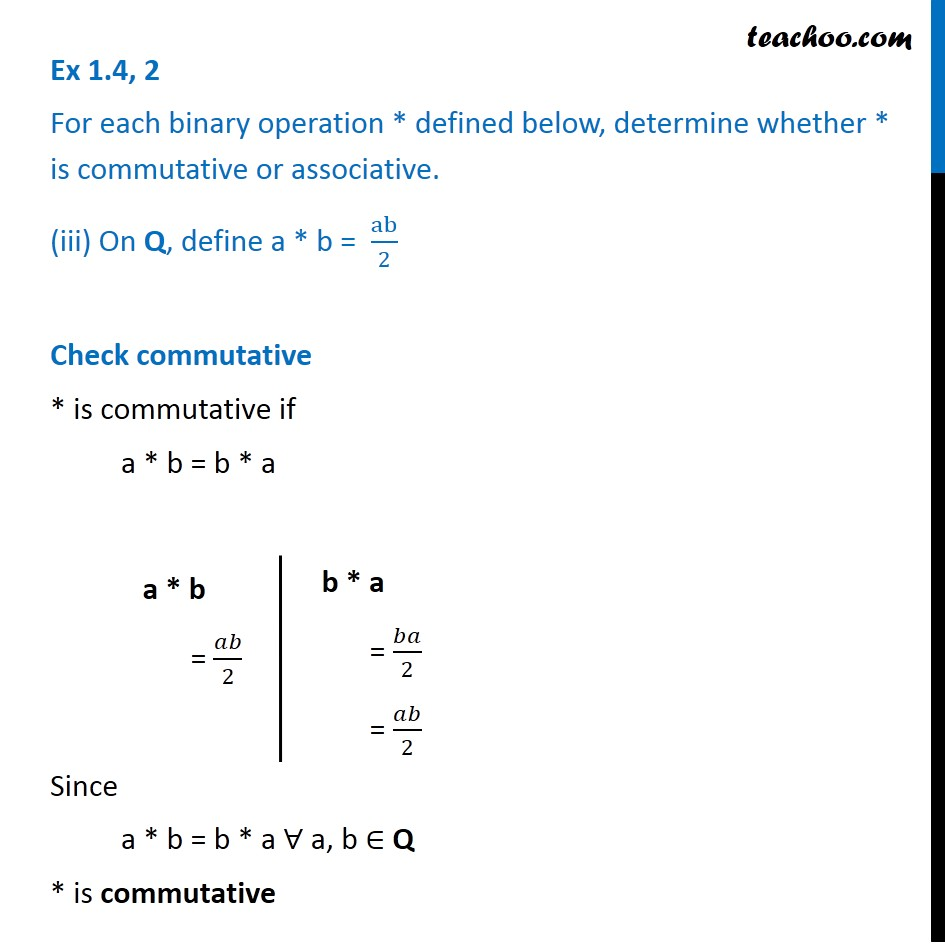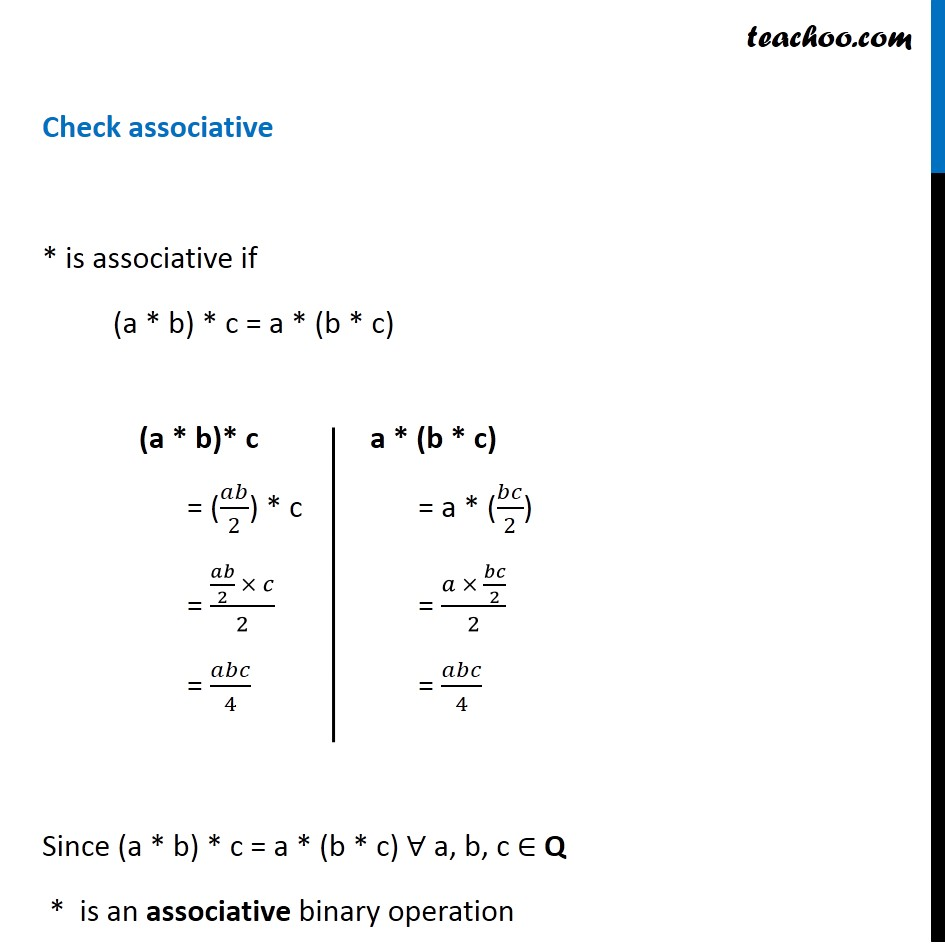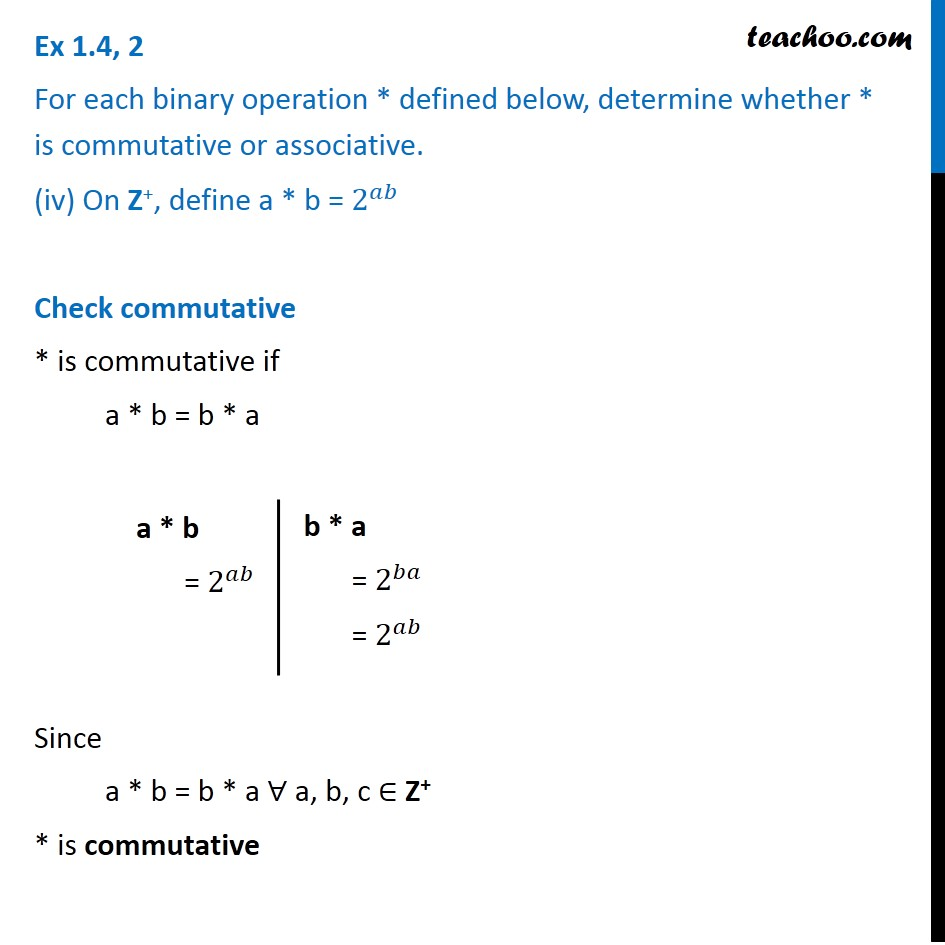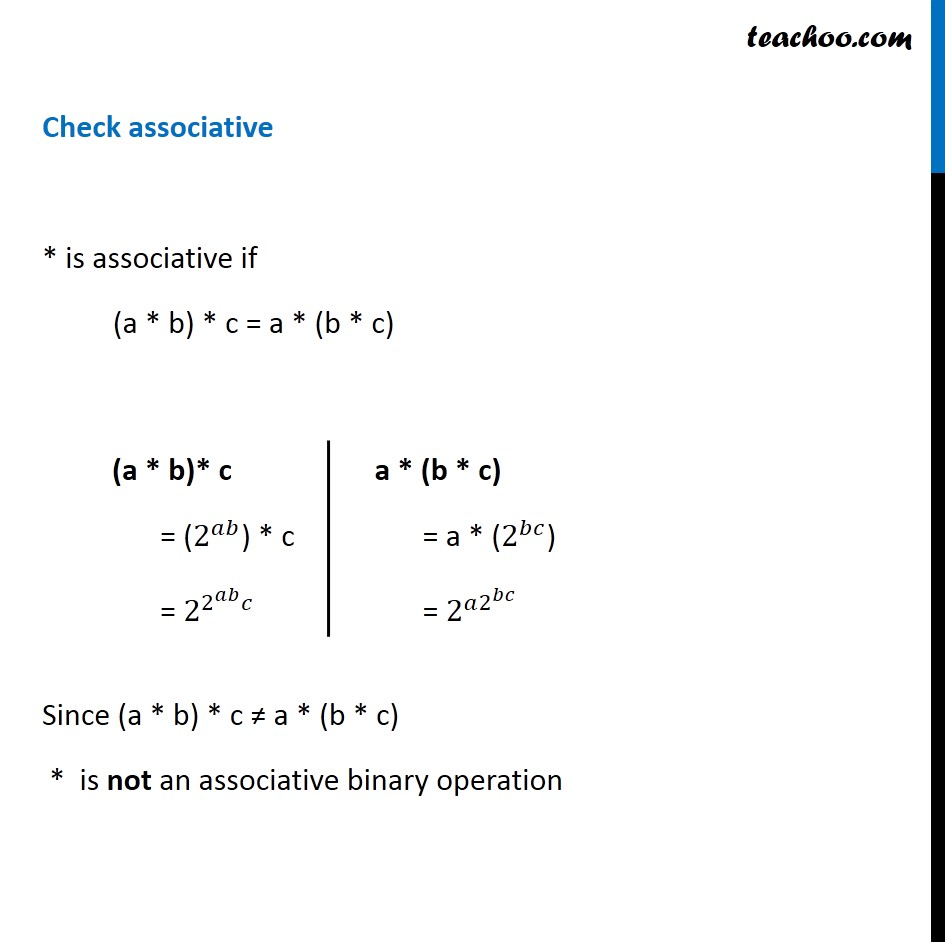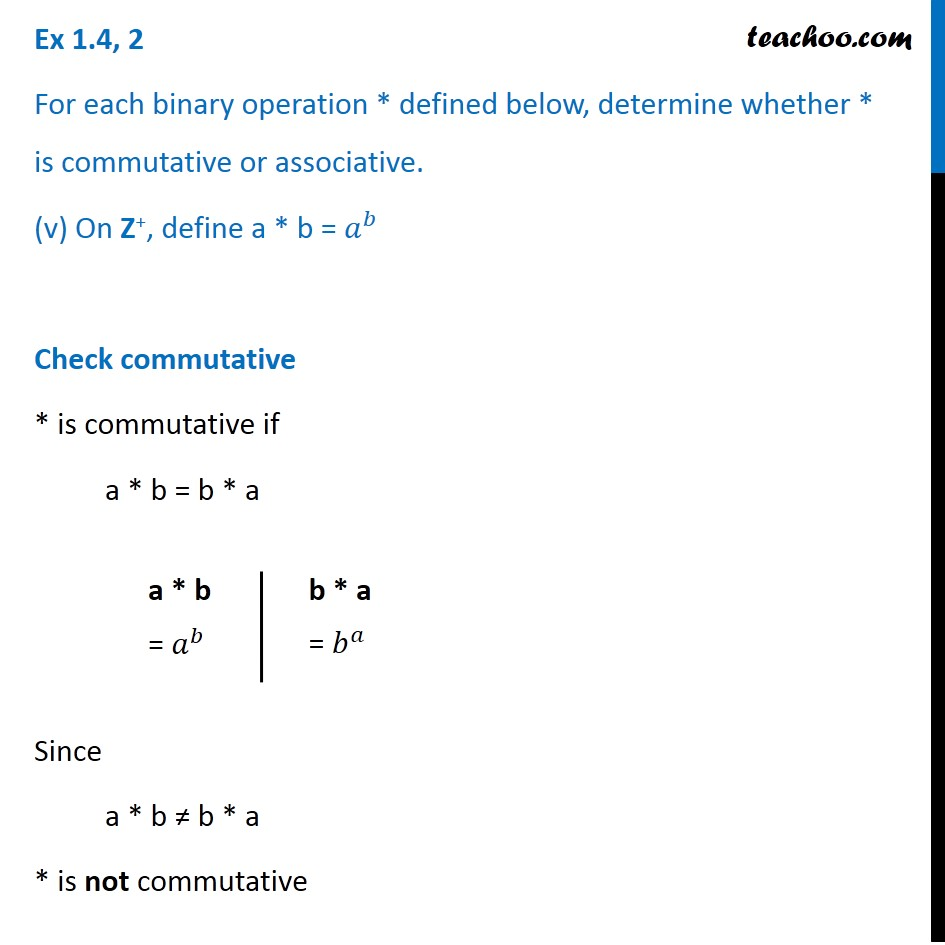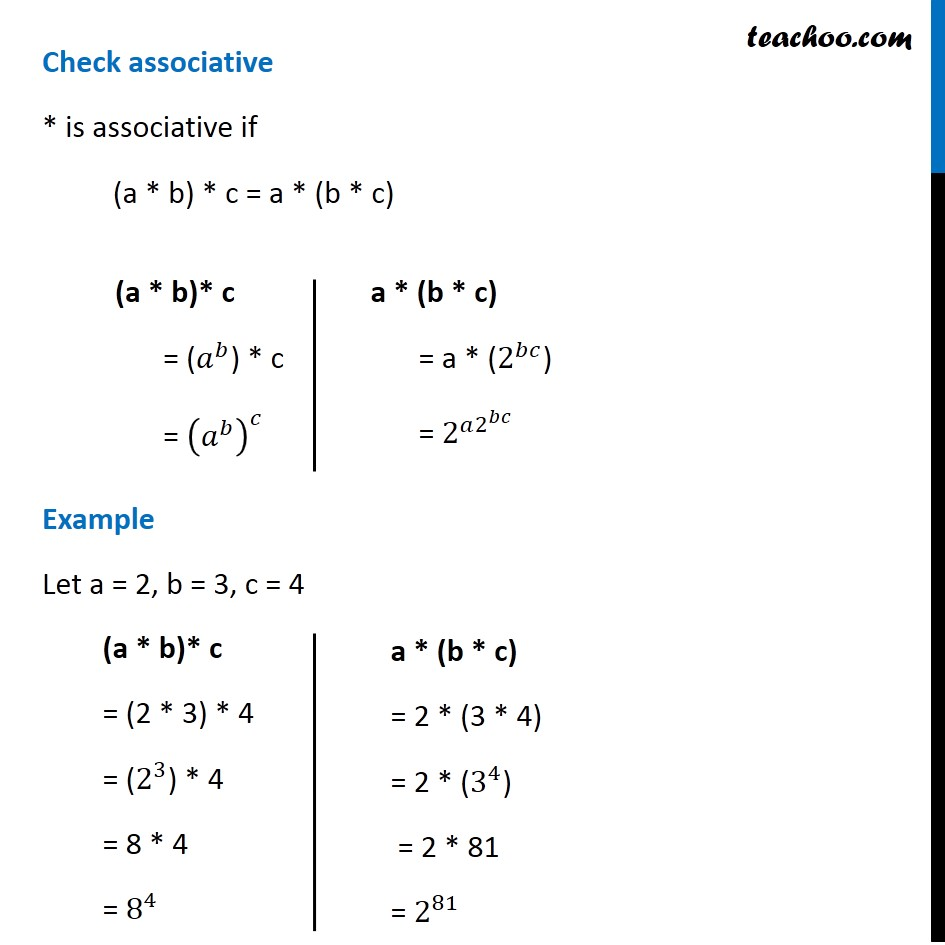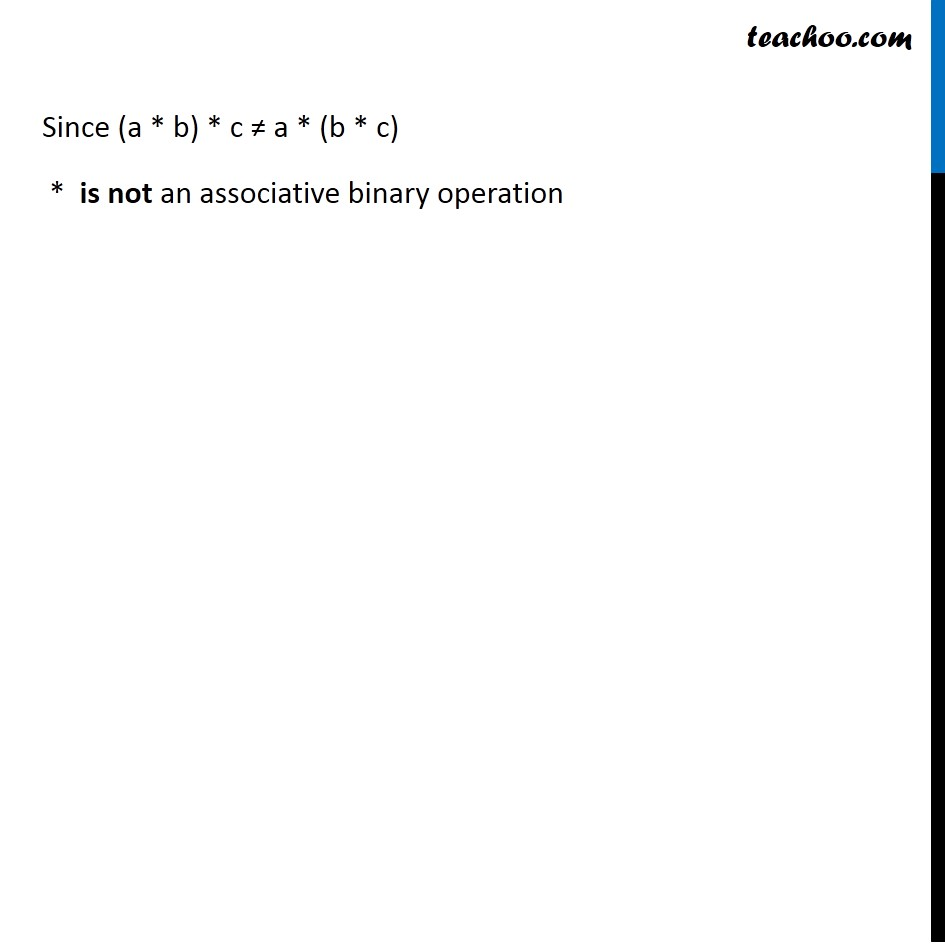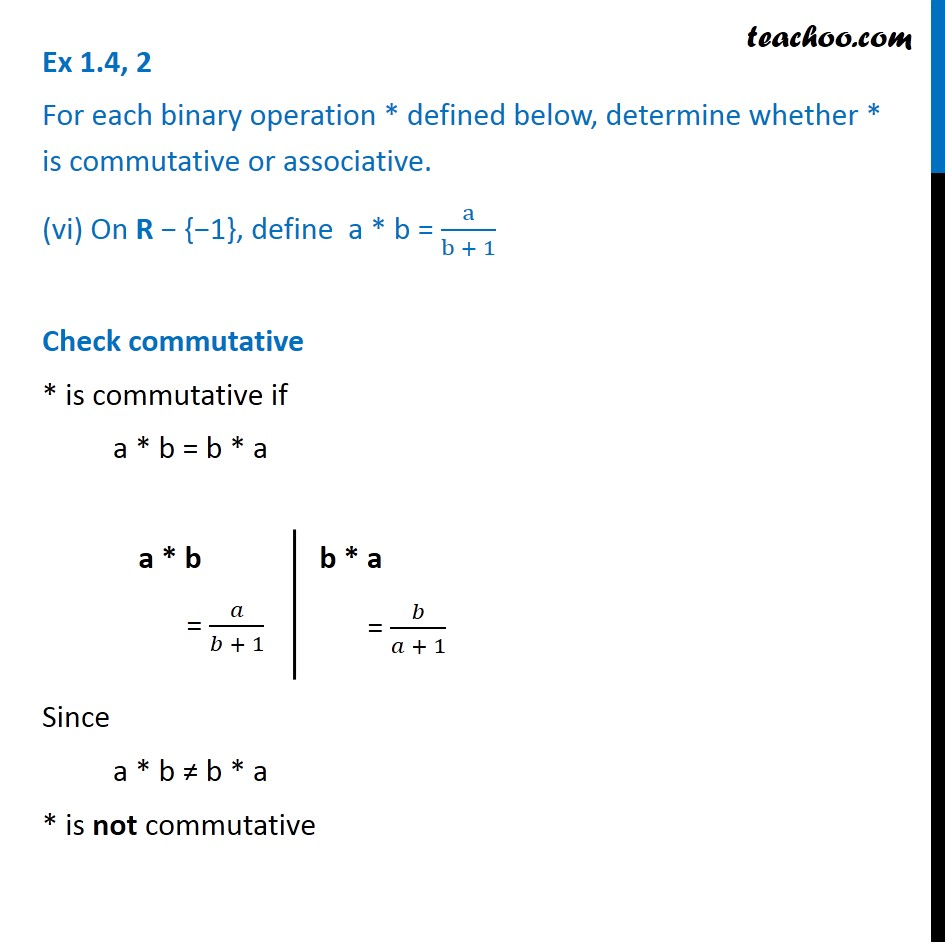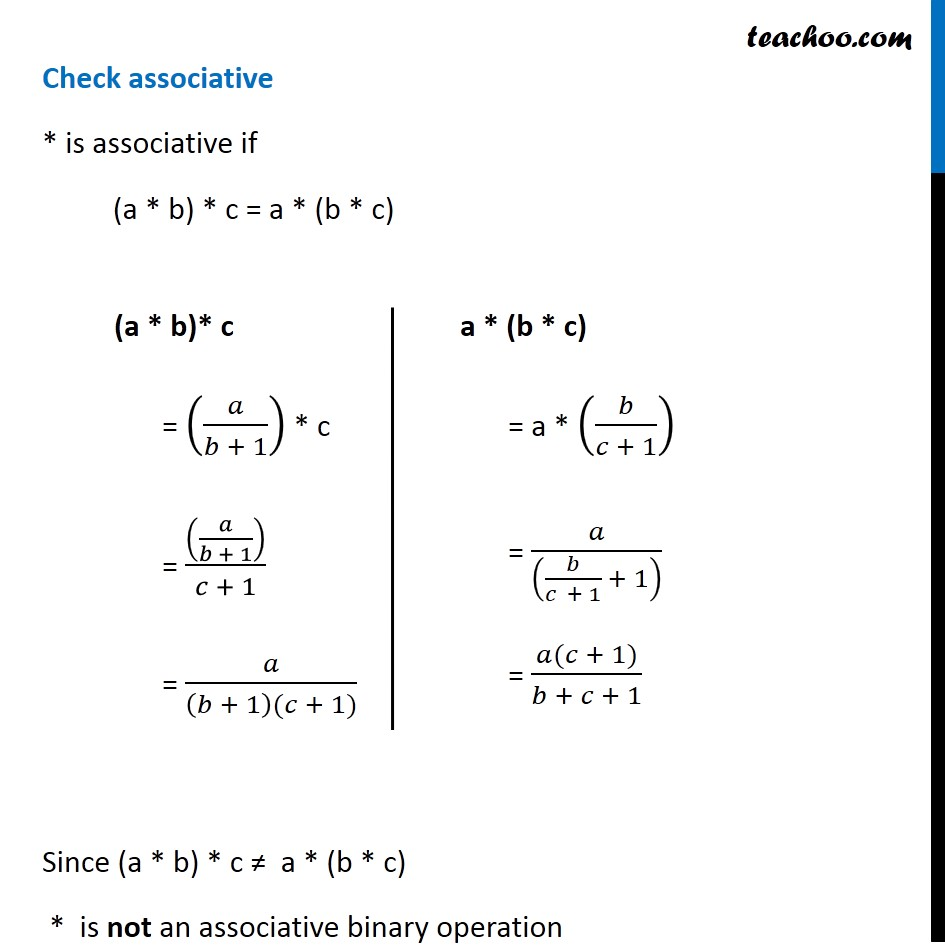Subscribe to our Youtube Channel - https://you.tube/teachoo

1. Chapter 1 Class 12 Relation and Functions
2. Serial order wise
3. Ex 1.4

Transcript

Ex 1.4, 2 For each binary operation * defined below, determine whether * is commutative or associative. (i) On Z, define a * b = a − b Check commutative * is commutative if a * b = b * a Since a * b ≠ b * a * is not commutative a * b = a – b b * a = b – a Check associative * is associative if (a * b) * c = a * (b * c) Since (a * b) * c ≠ a * (b * c) * is not an associative binary operation (a * b)* c = (a – b) * c = (a – b) – c = a – b – c a * (b * c) = a * (b – c) = a – (b – c) = a – b + c Ex 1.4, 2 For each binary operation * defined below, determine whether * is commutative or associative. (ii) On Q, define a * b = ab + 1 Check commutative * is commutative if a * b = b * a Since a * b = b * a ∀ a, b ∈ Q * is commutative a * b = ab + 1 b * a = ba + 1 = ab + 1 Check associative * is associative if (a * b) * c = a * (b * c) Since (a * b) * c ≠ a * (b * c) * is not an associative binary operation (a * b)* c = (ab + 1) * c = (ab + 1)c + 1 = abc + c + 1 a * (b * c) = a * (bc + 1) = a(bc + 1) + 1 = abc + a + 1 a * (b * c) = a * (bc + 1) = a(bc + 1) + 1 = abc + a + 1 Ex 1.4, 2 For each binary operation * defined below, determine whether * is commutative or associative. (iii) On Q, define a * b = ab/2 Check commutative * is commutative if a * b = b * a Since a * b = b * a ∀ a, b ∈ Q * is commutative a * b = 𝑎𝑏/2 b * a = 𝑏𝑎/2 = 𝑎𝑏/2 Check associative * is associative if (a * b) * c = a * (b * c) Since (a * b) * c = a * (b * c) ∀ a, b, c ∈ Q * is an associative binary operation (a * b)* c = (𝑎𝑏/2) * c = (𝑎𝑏/2 × 𝑐)/2 = 𝑎𝑏𝑐/4 a * (b * c) = a * (𝑏𝑐/2) = (𝑎 × 𝑏𝑐/2)/2 = 𝑎𝑏𝑐/4 Ex 1.4, 2 For each binary operation * defined below, determine whether * is commutative or associative. (iv) On Z+, define a * b = 2^𝑎𝑏 Check commutative * is commutative if a * b = b * a Since a * b = b * a ∀ a, b, c ∈ Z+ * is commutative a * b = 2^𝑎𝑏 b * a = 2^𝑏𝑎 = 2^𝑎𝑏 Check associative * is associative if (a * b) * c = a * (b * c) Since (a * b) * c ≠ a * (b * c) * is not an associative binary operation (a * b)* c = (2^𝑎𝑏) * c = 2^(2^𝑎𝑏 𝑐) a * (b * c) = a * (2^𝑏𝑐) = 2^(𝑎2^𝑏𝑐 ) Ex 1.4, 2 For each binary operation * defined below, determine whether * is commutative or associative. (v) On Z+, define a * b = 𝑎^𝑏 Check commutative * is commutative if a * b = b * a Since a * b ≠ b * a * is not commutative a * b = 𝑎^𝑏 b * a = 𝑏^𝑎 Check associative * is associative if (a * b) * c = a * (b * c) Example Let a = 2, b = 3, c = 4 (a * b)* c = (𝑎^𝑏) * c = (𝑎^𝑏 )^𝑐 a * (b * c) = a * (2^𝑏𝑐) = 2^(𝑎2^𝑏𝑐 ) (a * b)* c = (2 * 3) * 4 = (2^3) * 4 = 8 * 4 = 8^4 a * (b * c) = 2 * (3 * 4) = 2 * (3^4) = 2 * 81 = 2^81 Since (a * b) * c ≠ a * (b * c) * is not an associative binary operation Ex 1.4, 2 For each binary operation * defined below, determine whether * is commutative or associative. (vi) On R − {−1}, define a * b = a/(b + 1) Check commutative * is commutative if a * b = b * a Since a * b ≠ b * a * is not commutative a * b = 𝑎/(𝑏 + 1) b * a = 𝑏/(𝑎 + 1) Check associative * is associative if (a * b) * c = a * (b * c) Since (a * b) * c ≠ a * (b * c) * is not an associative binary operation (a * b)* c = (𝑎/(𝑏 + 1)) * c = ((𝑎/(𝑏 + 1)))/(𝑐 + 1) = 𝑎/((𝑏 + 1)(𝑐 + 1)) a * (b * c) = a * (𝑏/(𝑐 + 1)) = 𝑎/((𝑏/(𝑐 + 1) + 1) ) = (𝑎(𝑐 + 1))/(𝑏 + 𝑐 + 1)

Ex 1.4